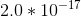Physical Chemistry : Equilibrium Constant and Reaction Quotient

Example Questions

Example Question #1 : Equilibrium Constant And Reaction Quotient

Consider the following reaction.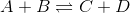A researcher adds equal volumes of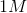substance A and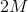substance B together. What is the ratio of concentration of C to concentration of D at equilibrium?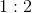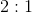Cannot be determined from the given information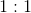Explanation:

To solve this question we need to use the definition of equilibrium constant. The equilibrium constant,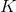, for this reaction is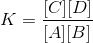For a givenvalue, the concentration of C and D would be the same. The stoichiometric coefficients of C and D are both 1; therefore, the amount of C and D produced would be the same and would depend on the limiting reagent. We do not know what the limiting reagent is (it could be A or B) and cannot determine the absolute concentrations of C and D; however, we can determine the relative concentrations. The concentration of C and D are the same and the ratio is 1:1.

Example Question #2 : Equilibrium Constant And Reaction Quotient

The reaction quotient of a reaction is twice as much as its equilibrium constant. What can you conclude about this reaction?

The forward reaction is occurring at a faster rate

The reverse reaction is occurring at a faster rate

Both forward and reverse reactions are occurring at a faster rate

Both forward and reverse reactions are occurring at a slower rate

The forward reaction is occurring at a faster rate

Explanation:

The reaction quotient,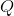, is calculated the same way as equilibrium constant; however, the concentrations used are derived from a nonequilbrium state. Consider the reaction below.If this reaction is NOT in equilibrium, then the reaction quotient is defined as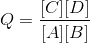If it’s at equilibrium then equilibrium constant is defined asNote that reaction quotient cannot be calculated when reaction is in equilibrium.

The question states that the reaction quotient is twice as much as the equilibrium constant. Since we can calculate reaction quotient, this reaction is not in equilbrium. The numerator foris higher than the numerator of(because reaction quotient is higher). There are more products created than reactants in the current nonequilbrium state and, therefore, the forward reaction is happening faster than the reverse reaction.

Example Question #141 : Physical Chemistry

Which of the following is true about equilibrium?

I. Enzymes increase the equilibrium constant

II. Equilibrium constant is only dependent on temperature

III. At equilibrium, there are no new products produced

I and III

II only

I only

II and III

II only

Explanation:

Chemical equilibrium is the state during which the rate of forward reaction equals the rate of reverse reaction. Equilibrium properties of a reaction determine how much products is produced. It’s important to remember that enzymes alter the rate of a reaction; however, they DO NOT alter the equilibrium. This means that enzymes speed up a reaction; however, they do not increase the amount of products produced.

ONLY increasing or decreasing temperature will alter equilibrium constant. Other factors such as concentration, volume, and pressure do not change it. Changing these other factors might shift the reaction left or right to bring the reaction back to equilibrium; however, it does not change the equilibrium constant. This means that these other factors can change the individual concentration of reactants and products at equilibrium, but they will not change the ratio of products to reactants.

As mentioned, at equilibrium the rates of forward and reverse reactions are equal. This means that new products and reactants are constantly being produced; however, there is no net production of these molecules.

Example Question #4 : Equilibrium Constant And Reaction Quotient

According to Le Chatelier’s principle, the reaction will shift to the right (towards the products) when the __________ is less than __________.

equilibrium constant . . . activation energy

reaction quotient . . . equilibrium constant

reaction quotient . . . activation energy

equilibrium constant . . . reaction quotient

reaction quotient . . . equilibrium constant

Explanation:

Le Chatelier’s principle states that a chemical system will respond to changes in the environment and maintain equilibrium by changing the direction of reaction. A reaction will shift to the right if the ratio of concentration of products to reactants goes down and will shift to the left if the ratio goes up. Reaction quotient,, characterizes the state of a reaction in a nonequilbrium state. It is calculated the same way as equilibrium constant.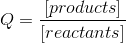The question is asking about a shift to the right. Reaction shifts to the right (and approaches equilbrium) when the nonequilbrium reaction has more reactants than products. This occurs when the ratio of products to reactants () is lower than the ratio of products to reactants at equilibrium ().

Example Question #5 : Equilibrium Constant And Reaction Quotient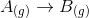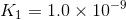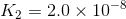What is the value of the equilibrium constant for the following reaction?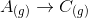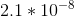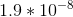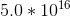There is not enough information to answer this question